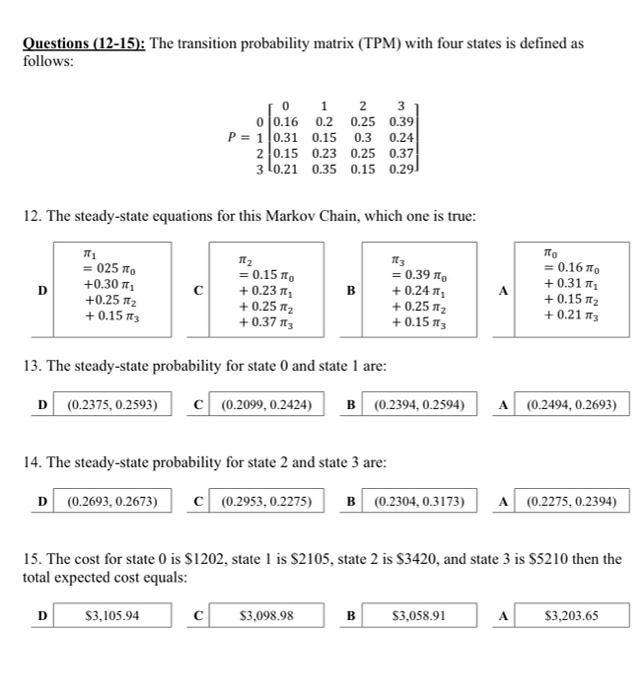# (Solved): Please solve transition probability matrix (TPM) problems thanks Questions (12-15): The transition p ...

Please solve transition probability matrix (TPM) problems thanksQuestions (12-15): The transition probability matrix (TPM) with four states is defined as follows: $P=\begin{array}{r} 0 \\ 1 \\ 2 \end{array}\left[\begin{array}{cccc} 0 & 1 & 2 & 3 \\ 0.16 & 0.2 & 0.25 & 0.39 \\ 0.31 & 0.15 & 0.3 & 0.24 \\ 0.15 & 0.23 & 0.25 & 0.37 \\ 0.21 & 0.35 & 0.15 & 0.29 \end{array}\right]$ 12. The steady-state equations for this Markov Chain, which one is true: \begin{tabular}{|ll} \hline & $$\pi_{0}$$ \\ & $$=0.16 \pi_{0}$$ \\ & $$+0.31 \pi_{1}$$ \\ & $$+0.15 \pi_{2}$$ \\ & $$+0.21 \pi_{3}$$ \\ \hline \end{tabular} 13. The steady-state probability for state 0 and state 1 are: 1 C A 14. The steady-state probability for state 2 and state 3 are: D A 15. The cost for state 0 is $$\ 1202$$, state 1 is $$\ 2105$$, state 2 is $$\ 3420$$, and state 3 is $$\ 5210$$ then the total expected cost equals: D

We have an Answer from Expert